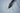# Rules of ExponentsA mathematical operation that is written as my, which involves two numbers, x known as the base and y known as the exponent or power.

It is said that m is raised to the power of y and this process is called raising to a power. Thus, we can say that the repeated multiplication of the same thing or the base is called exponents. my is the product of multiplying y bases. For example, 23. We say that 2 is raised to the power of 3. 23 means that 2 is multiplied 3 times, i.e., 2 * 2 * 2 = 8.

Hence, the value of 23 is 8. In this article, we will take a look at the rules that are used to perform different exponent operations.

## Product Rule:

This rule applies to the product of two exponents with the same bases. It states that for any non zero term m, ma × mb = ma + b

where a and b are real numbers. If we have two exponents with different bases but the same exponent then this rule is given as ma × na = (mn)a

## Quotient Rule:

The quotient rule applies to exponents where the bases are the same.

ma/mb = ma – b

When the exponents have different bases with the same power then, ma/na = (m / n)a. Where a and b are real numbers and m is a non zero term.

## Power Raised to Power Rule:

If m is the base, and it is raised to a power a, and this entire expression is raised to another b then this term is equivalent to the product or the powers raised to the base m. This is given as

(ma)b = mab

## Negative Exponentiation Rule:

If ‘a’ base is ‘m’ is raised to an exponent that has a negative value, then this exponent can be changed to a positive value by using a reciprocal expression. This is denoted as follows.

m-a = 1/ma. where m is a non-zero term, and a is a real number.

## Zero Rules:

When any base, irrespective of its value, is raised to the power of 0 then the result of the exponent is 1. m0 = 1.

## One Rule:

If 1 is raised to an exponent, the resultant will always be 1. This is given as: 1m = 1

###### Your Ultimate Checklist To Prep For A CPA Exam

Suppose we are dealing with the negative integer -1, then this rule is modified as follows:

• (-1)n = 1, where n is an even whole number.
• (-1)n = -1, where n is an odd whole number.

## Fractional Rule:

If a base m is raised to a fractional power (1/a), then it can be expressed as the ath root of that number as follows: m1/a =

Further, if the base is raised to a fractional power where the numerator is greater than 1 then, the exponent can be expressed as follows: mb/a =

Thus, these are the exponent rules that are used widely in simplifying and solving problems.

## Conclusion:

Exponents can prove to be a confusing topic as there are too many rules. Thus, kids should approach an educational platform such as Cuemath to help them in their understanding of this subject.

At Cuemath, the math experts teach students using several resources like worksheets, math puzzles, games, visual simulations, and apps to ensure that they have a holistic development environment. Kids are also encouraged to follow their learning styles and can master the subject in no time.